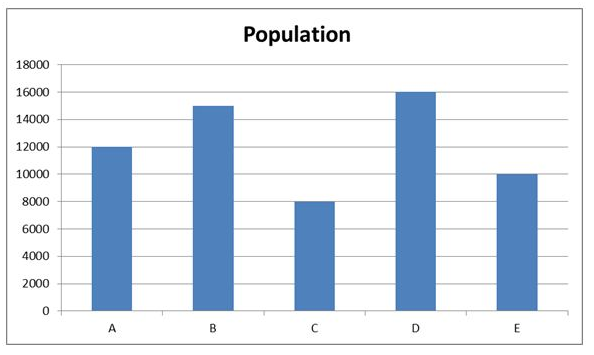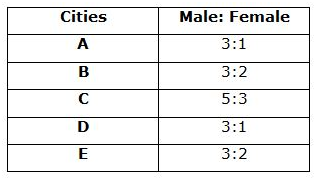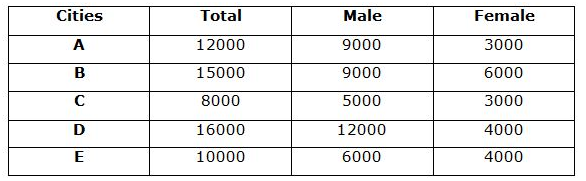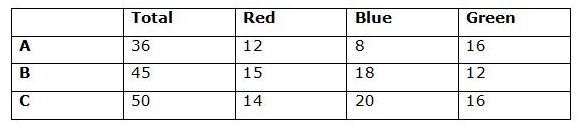# IBPS PO Prelims Quantitative Aptitude (Day-08)

Dear Aspirants, Our IBPS Guide team is providing new series of Quantitative Aptitude Questions for IBPS PO Prelims 2020 so the aspirants can practice it on a daily basis. These questions are framed by our skilled experts after understanding your needs thoroughly. Aspirants can practice these new series questions daily to familiarize with the exact exam pattern and make your preparation effective.

Start Quiz

Data Interpretation

Direction (1-6): Study the following information carefully and answer the questions given below.

The given bar graph shows the number of population in five different cities – A, B, C, D and E in 2018The given table shows the number of male to female population in five different cities.1) What is the total number of female population in City D and E together?

A) 4000

B) 6000

C) 8000

D) 10000

E) None of these

2) What is the average number of male population in all the cities together?

A) 7600

B) 7900

C) 8200

D) 8500

E) None of these

3) What is the difference between the number of female population in B and E together and the number of female population in A and C together?

A) 5000

B) 4000

C) 3000

D) 2000

E) None of these

4) If the ratio of the number of population in below poverty to above poverty in city B is 13: 17, then what is the difference between the number of male population from A and the number of above poverty population in B?

A) 300

B) 400

C) 500

D) 200

E) None of these

5) In 2019 the number of female population in D is increased by 40% compared to previous year and the number of male population in D is decreased by 20% compared to previous year. What is the total number of population in D in 2019?

A) 15200

B) 15600

C) 14900

D) 14960

E) None of these

6) What is the ratio of the number of male population in C and E together to the average number of female population from A, B, D and E together?

A) 38:11

B) 39:16

C) 40:13

D) 44:17

E) None of these

Caselet

Direction (7-9): Study the following information carefully and answer the questions given below.

Box A: The total number of balls is 36 and the ratio of the number of red, blue and green balls in box A is 3:2:4.

Box B: The total number of balls is 25% more than that of the number of balls in box A. The number of red balls in box B is 25% more than that of the number of red balls in A. The ratio of the number of blue to green ball in B is 3:2

Box C: Total number of balls is C is 5 balls more than that of the total number of balls in B. If the number of red balls in C is one less than that of the number of red balls in B. The number of blue balls in C is sum of the red and blue balls in A.

7) What is the total number of green balls in all the boxes together?

A) 42

B) 44

C) 48

D) 41

E) 44

8) What is the ratio of the total number of red balls in all the boxes together to the total number of balls in box B?

A) 1: 5

B) 11: 15

C) 14: 15

D) 7: 9

E) None of these

9) The total number of blue balls in all the boxes together is what percent of the total number of balls in box C?

A) 95%

B) 93%

C) 90%

D) 91%

E) 92%

Following questions contain two statements as statement I and statement II. You have to determine which statement/s is/are necessary to answer the question and give answer as,

10) What is the perimeter of the rectangle?

Statement I: If the length of the rectangle is decreased by 3 cm and breadth of the rectangle is increased by 5 cm, the area of the rectangle is 117 cm2.

Statement II: Length of the rectangle is 4 cm less than that of the breadth after the changes.

A) The data in statement I alone is sufficient to answer the question, while the data in statement II alone is not sufficient to answer the question

B) The data in statement II alone is sufficient to answer the question, while the data in statement I alone is not sufficient to answer the question

C) The data either in statement I alone or in statement II alone is sufficient to answer the question

D) The data given in both statements I and II together are not sufficient to answer the question and

E) The data given in both statements I and II together are necessary to answer the question.

Directions (1-6):Required total = 4000 + 4000 = 8000

Required Average = (9000 + 9000 + 5000 + 12000 + 6000)/5 = 8200

B and E female population = 6000 + 4000 = 10000

A and C female population = 3000 + 3000 = 6000

Difference = 10000 – 6000 = 4000

Number of above poverty population in B = 17/30 * 15000 = 8500

Number of male population in A = 9000

Difference = 9000 – 8500 = 500

Number of female population in D in 2019 = 4000 * 140/100 = 5600

Number of male population in D in 2019 = 12000 * 80/100 = 9600

Total population in D in 2019 = 5600 + 9600 = 15200

Required ratio = (5000 + 6000):(3000 + 6000 + 4000 + 4000)/4

= 11000:4250

= 220:85

= 44:17

Directions (7-9):

Box A:

Total = 36

Red = 36 * 3/9 = 12

Blue = 36 * 2/9 = 8

Green = 36 * 4/9 = 16

Box B:

Total = 125/100 * 36 = 45

Red = 125/100 * 12 = 15

Blue = (45 – 15) * 3/5 = 18

Green = 30 * 2/5 = 12

Box C:

Total = 45 + 5 = 50

Red = 15 – 1 = 14

Blue = 12 + 8 = 20

Green = 50 – 14 – 20 = 16Total = 16 + 12 + 16 = 44

Required ratio = (12 + 15 + 14):45

= 41: 45

Required percentage = [(8 + 18 + 20)/50] * 100

= 92%

From statement I,

(l – 3) * (b + 5) = 117

So, Statement I alone is not sufficient to answer the question.

From statement II,

l – 3 + 4 = b + 5

l = 4 + b

So, Statement II alone is not sufficient to answer the question.

From I and II

(l – 3) * (b + 5) = 117

(4 + b – 3) * (b + 5) = 117

(b + 1) * (b + 5) = 117

b2 + 6b – 112 = 0

Solving this,

b = -14, 8

b = 8 (neglecting –ive value)

l = 8 + 4 = 12

Area = lb = 12 * 8 = 96

Both statements are necessary to answer the question.

 Check Here to View IBPS PO Prelims 2020 Quantitative Aptitude Questions Day – 07 Day – 06 Day – 05